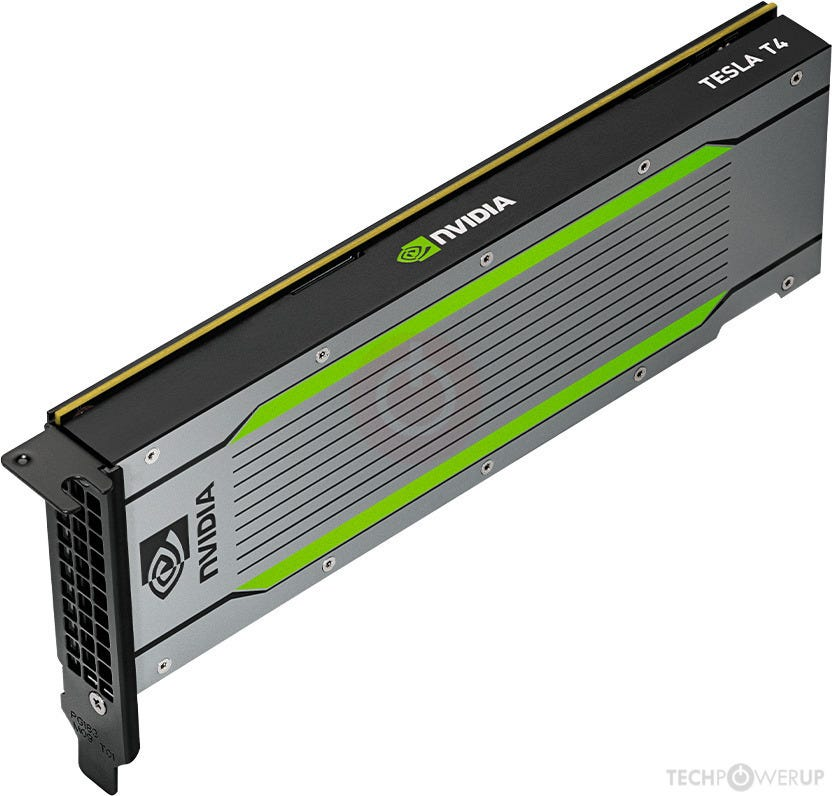# Tutorial 9: TPU vs. GPU

Source: Deep Learning on Medium

Prerequisite: Tutorial 1 (MNIST) and Tutorial 2 (Cifar10)

In late April 2019, Google upgraded the GPU in Colab from the outdated Tesla K80 to the much newer Tesla T4. This is a data center GPU with no fan — I guess it must be very quiet.Tesla T4: a thin, quiet GPU with no fan. © TechPowerUp

T4 is 4 generations ahead of K80: after K (Kepler) there is M (Maxwell), and then P (Pascal), and then V (Volta), and finally T (Turin). How does the T4 compare with Colab’s TPU? For single-precision float number operations, T4 is only 8.1 tflops, compared to the TPU’s 45 tflops per chip. How about their practical performance? Let’s find out.

The first step is to set the Colab hardware accelerator to “GPU”, and check the specs:

`!nvidia-smi`

So, this is a T4 GPU with 15GB memory. Now let’s test it with our first tutorial on MNIST, and see how fast it is. With Fenwicks, the code is almost exactly the same as on the TPU. The only difference is that the GPU, being a card within the Colab’s machine, can directly write data to its hard drive. This means there’s no longer any need to use Google Cloud Storage. So we should kick out the line for setting up GCS.

Apart from deleting the GCS set up code, everything else is the same. Here’s the Jupyter notebook:

One thing that is a bit surprising is the result accuracy: only 99.2%. If you repeat the code several times, you can occasionally get 99.4%, but not frequently. In contrast, on the TPU you usually see 99.4%. But this is exactly the same code! What’s going on?

Let’s test our code for another dataset: Cifar10. We start with the code from Tutorial 2, and remove the line to set up GCS. However, this time we get an error: that the code runs out of memory during evaluation. Recall that for model evaluation, put the entire validation set in one batch, which needs a lot of memory. But, we didn’t get any complaint from the TPU, why does the GPU run out of memory?

The reason is that the TPU is in a pod somewhere else, unlike the GPU, which is inside Colab’s machine. So, to use the TPU, we have to access it through the network. After training our model, the TPU is disconnected and closed, and its memory is cleared. When we evaluate the model, we re-connect to the TPU, which has a clean memory, which is sufficient to hold the entire validation set.

The GPU, on the other hand, doesn’t release its memory after model training. So training specific variables, such as the momentum values for the Adam optimizer (two variables for every model parameter), stay in memory. As a result, there’s much less memory left for evaluation. To fix the out-of-memory error, we use a smaller validation batch size:

`VALID_BATCH_SIZE = 1000`

And use this batch size when creating our TPUEstimator:

`est = fw.train.get_tpu_estimator(steps_per_epoch, model_func, work_dir, trn_bs=BATCH_SIZE, val_bs=VALID_BATCH_SIZE)`

The evaluation now takes more than 1 step:

`result = est.evaluate(eval_input_func, steps=n_test // VALID_BATCH_SIZE)`

Now let’s run the code again. This time it runs smoothly. The result? Again, slightly worse than on the TPU: only 92% rather than 94% as in Tutorial 2.

Remember that Tutorial 2 basically re-implement the DavidNet model, and modified one hyperparameter: the weight decay. In DavidNet, the weight decay factor is 0.0005, which is a common value originating from AlexNet, the mother of all deep learning models. On the TPU, however, this value appears too large, and our model underfit. So, we tuned it down to 0.000125, and the model reached 94%.

DavidNet was designed for the GPU, so its original hyperparameters should work on the Tesla T4. Let’s do that:

`WEIGHT_DECAY = 0.0005 #@param ["0.000125", "0.00025", "0.0005"] {type:"raw"}`

As expected, the model reaches 94% this time, though the code is around 5x slower than on the TPU. Here’s the Jupyter notebook:

So now we know: the GPU and TPU are very different devices that require different hyperparameters. What’s the reason for this difference? One main reason is that the TPU contains 8 cores, each processing 1/8 of a batch independently. This means that the TPU is not one device, but 8 — in a way, it’s similar to an array of 8 weaker GPUs, rather than a strong one.

Let’s do one more experiment to confirm this: in the GPU code, we use the TPU’s weight decay factor: 0.000125, and at the same time tune down the batch size from 512 to 512/8 = 64. Run it again. The model should reach 94%, or at least a high 93.X%. On the TPU, each of the 8 cores in fact handles 512/8=64 training records. This sheds light on the difference of hyperparameters.

Lastly, in the GPU code, let’s set the batch size to 128, and weight decay to 0.000125. This time, the code again easily reaches 94%. In the theory of deep learning training dynamics, a 4x drop in batch size (from 512 to 128) is roughly equivalent to increasing the learning rate by 4x. This cancels out the 4x drop in weight decay factor, as the weight decay factor is first multiplied with the learning rate inside the SGD optimizer.

All tutorials: